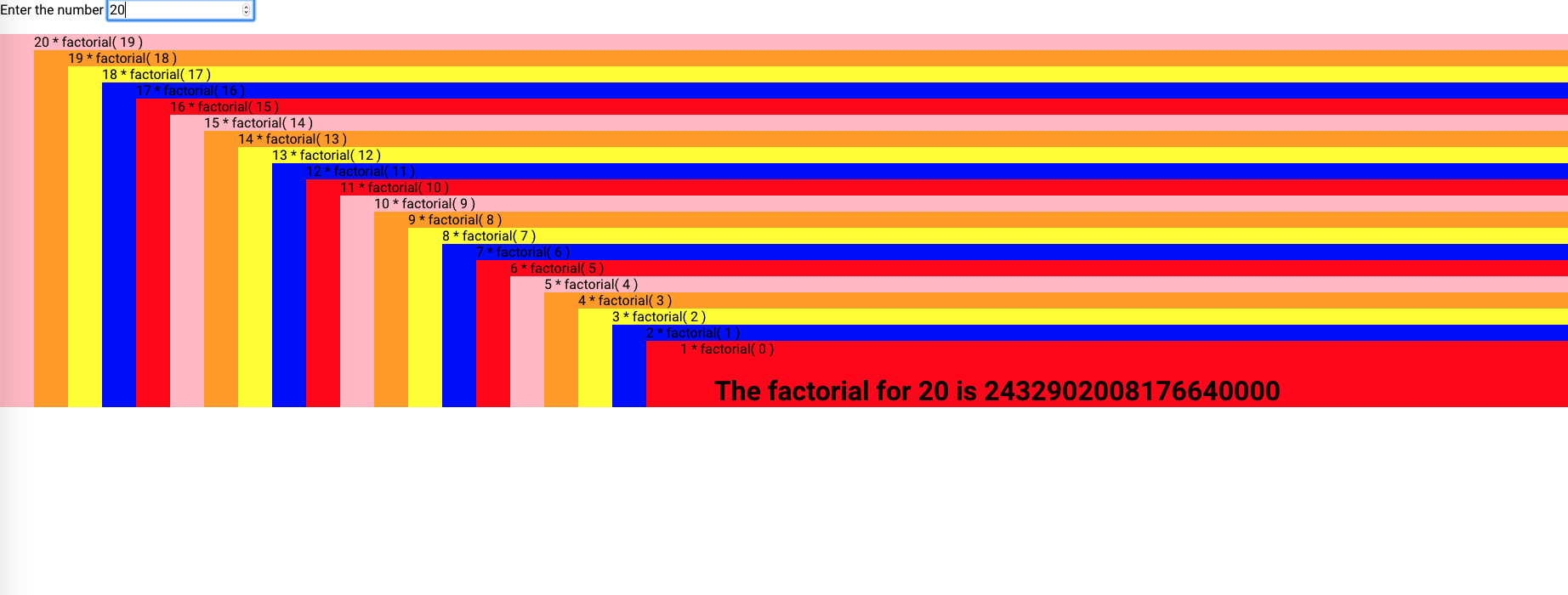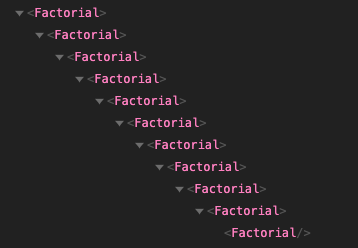# Factorial

The product of (= the result) a whole number and all the whole numbers below it

Four factorial (4 x 3 x 2 x 1) is 24

## Goal

Create a Factorial component which will calculate the answer recursively using only templates and helpers.

### Step 1 - How to calculate factorial using recursion

Let's first see how to solve the factorial using recursion in JS land

``````function factorial(number) {
if(number === 0) return 1; //when we get to the base case it returns 1.
return number * factorial(number-1) //Recursion, we are calling factorial again with n-1
}

factorial(3) //6
``````

### Step 2 - Factorial Component

Let's write the same, but using ember templates (this is not the final version), it's purpose is to show similarity with the js equivalent

eq is a helper to test equality `{{eq "hola" "bye"}} = false`

sub is a helper to subsctract something `{{sub 5 1}} = 4`

mult is a helper to multiply something `{{mult 5 2}} = 10`

You can find these helpers and more at

ember-math-helpers

ember-truth-helpers

``````{{! factorial.hbs}}
{{#if (eq @number 0)}}
{{return 1}} {{! when we get the base case, it retuns 1.}}
{{else}}
{{return
(mult @number <Factorial @number={{sub @number 1}} />)
}} {{! Recursion, we're calling factorial again with n-1}}
{{/if}}
``````

This algorithm seems correct, and conceptually this is the same as the `JS` equivalent, but it has some errors.

First, when you want to `return` something in ember templates, you use `yield` keyword instead of `return`

``````{{! factorial.hbs}}
{{#if (eq @number 0)}}
{{yield 1}} {{! when we get to the base case, return 1.}}
{{else}}
{{yield
(mult @number <Factorial @number={{sub @number 1}} />)
}} {{! Recursion, we're calling factorial again with n-1}}
{{/if}}
``````

By last, this is the difficult part where we find ourselves a bit lost, whereas it can actually yield or "return" a component

``````{{yield (component 'factorial' @number=(sub @number 1)}}
``````

This component would not actually run, so the client of this code would need to do something like this.

``````{{#let 10 as |number|}}
<Factorial @number={{number}} as |Factorial|>
<Factorial />
</Factorial>
{{/let}}
``````

Which actually does nothing because we are never getting the answer.

Here's the solution

``````{{! factorial.hbs}}
{{#if (eq @number 0)}}
{{yield 1}} {{! when we get to the base case, return 1.}}
{{else}}
{{! Recursion, we are calling factorial component again with n-1}}
<Factorial @number={{sub @number 1}} as |response|>
</Factorial>
{{/if}}
``````

By yielding the multiplication of the current number times the response of another factorial(n-1) (inside the block), we just covered the recursion.

Here's the final Component "API".

``````{{#let 10 as |number|}}
</Factorial>
{{/let}}
``````

Finally if we wish to visually display or render the recursive tree nicely, we could use the `<ul>` tag

``````{{! factorial.hbs}}
{{#let
(array "red" "blue" "yellow" "orange" "pink") as |colors|
}}
<ul style="background-color: {{object-at (mod (sub @number 1) colors.length) colors}};">
{{#if (eq @number 0)}}
{{yield 1}} {{! when we get to the base case, return 1.}}
{{else}}
{{@number}} * factorial({{sub @number 1}})
{{! Recursion, we are calling factorial component again with n-1}}
<Factorial @number={{sub @number 1}} as |response|>
</Factorial>
{{/if}}
</ul>
{{/let}}
``````And the Ember inspector would look like this!## Why using templates instead of pure JavaScript?

Ember templates rerender automatically when some value (must be a `@tracked` decorated property or an Ember.Object property) referenced in them, changes, this means that we could have an observed recursion. Our components tree can actually make smart decisions, so we can have a recursive logical tree that recalculates on arbitrary events, like clicking a button that might increment a property referenced by the template thus, triggering a rerender, etc. In other words, we can take advantage that ember templates already know exactly when to "rerender" as effective observer(s) of our recursion.

You can of course solve these kind of problems also by adding observers in your components js class, or some other technique, which is usually way more verbose and would need some kind of hand wiring observers via `addObserver` and then destroy them if the component gets destroyed.

Our use case was very strange and specific, we wanted a way to reduce a complex nested object (flatten an object) to create a live array, so a client could iterate on. This "live" stuff would use the templates "observavility" and automatic "rerenders" to effectively observe an external dependency, i.e. `DS.Model` instance, because the flattening process has logical branches based on the `DS.Model` properties actual values (referenced in the templates). Since any change in `DS.Model` would cause a complete rerender, and the performance implications were dire, we chose other path.